maths > wholenumbers

Subtraction: Simplified Procedure for Large Numbers

what you'll learn...

overview

The subtraction in first principle was explained as taking away part of a quantity and counting or measuring the remaining quantity.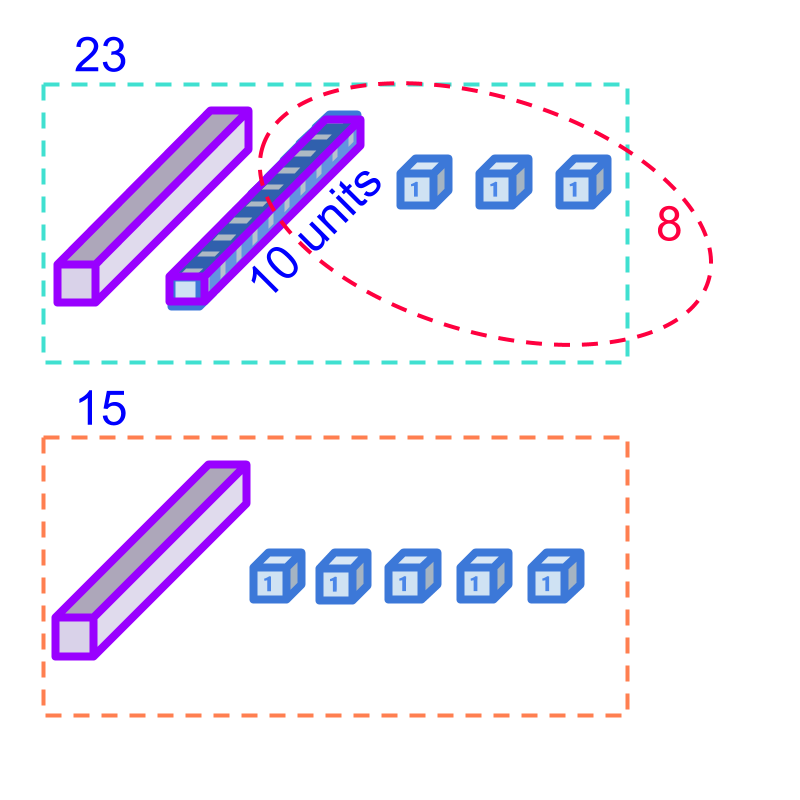This method is revised for 2-digit numbers and established that the tens place and units placed can be subtracted separately. This sets up learning subtraction by place-value.

In doing the subtraction by place-value, the minuend-digit can be smaller than the subtrahend-digit and that sets up learning de-grouping. The de-grouping is explained as the borrow in subtraction.

de-grouping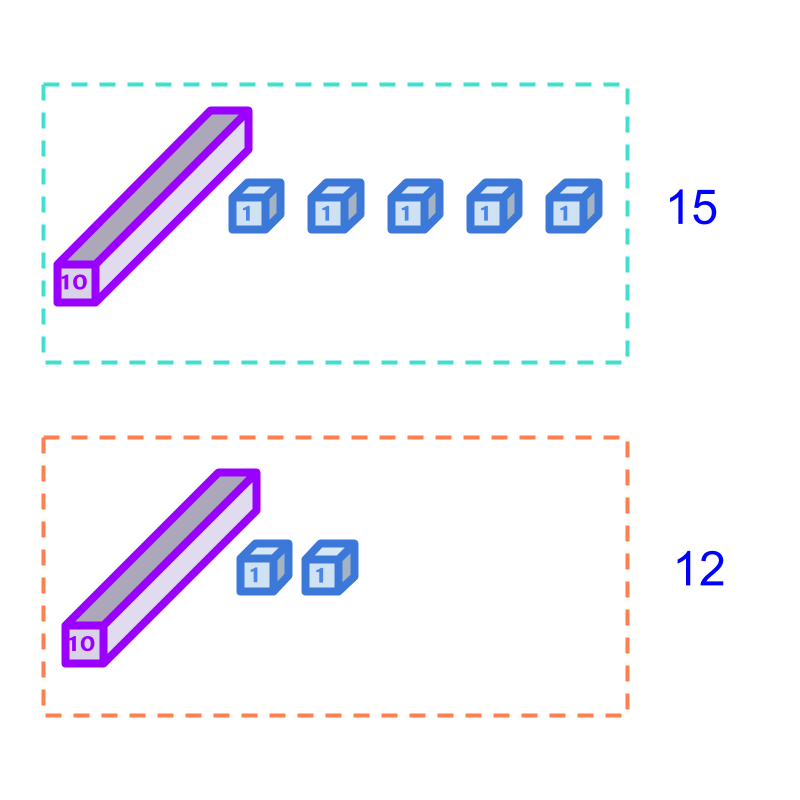Consider the subtraction $15-12$$15 - 12$. From 15, 12 is taken away. The remaining quantity is counted.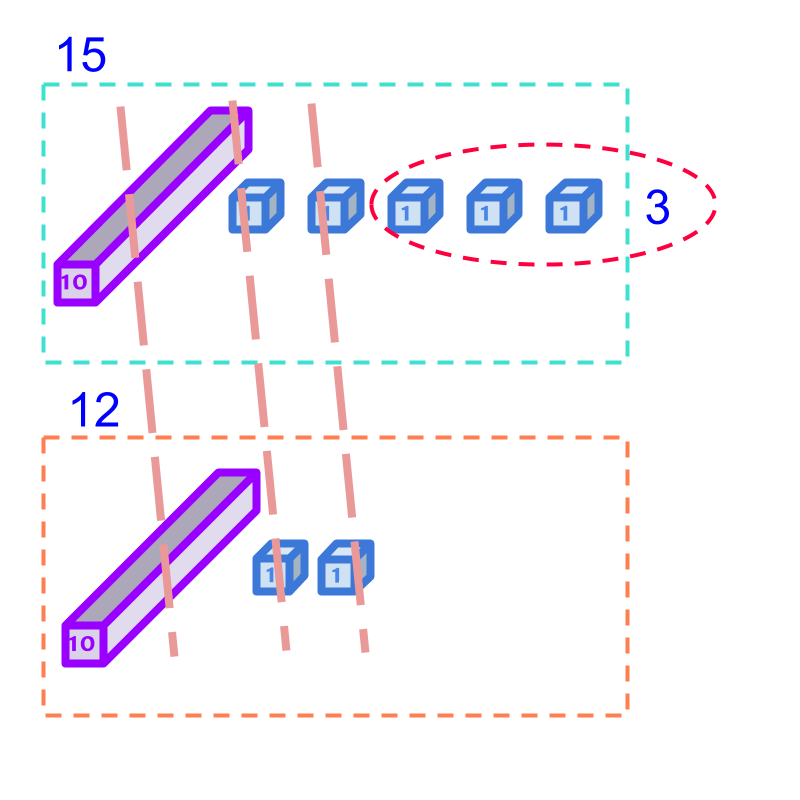Considering the subtraction $15-12$$15 - 12$. Taking away $12$$12$ from $15$$15$ is shown in the figure. The dotted lines cross the equal amount from the numbers $12$$12$ and $15$$15$. The remaining quantity is shown in the dotted ellipse. The count of remaining amount is $3$$3$.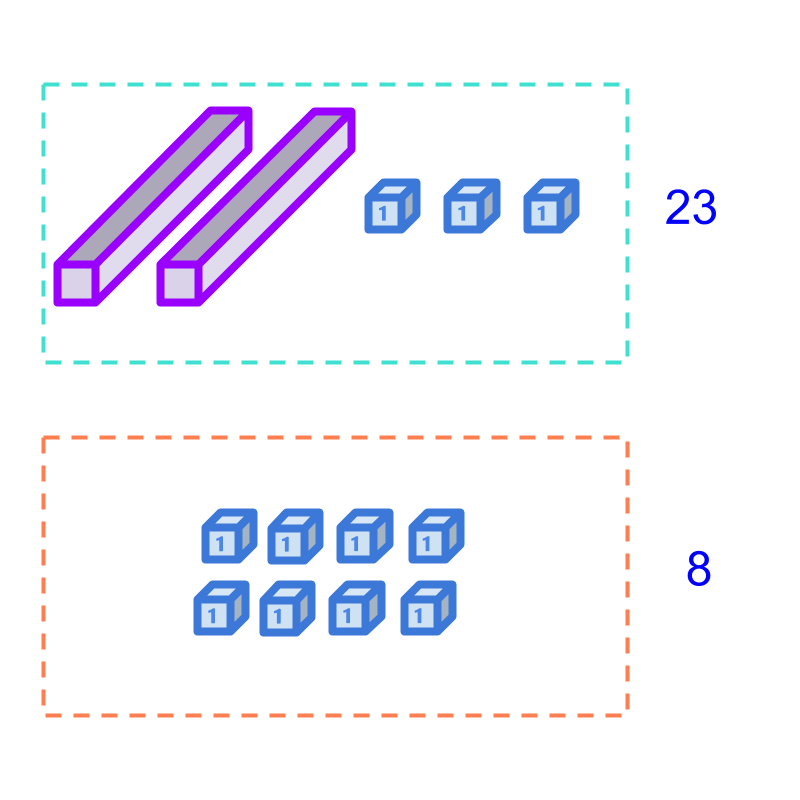Consider the subtraction $23-8$$23 - 8$. The quantities are shown in the figure. Taking away $8$$8$, from $23$$23$ is to be done. The $3$$3$ units in $23$$23$ is smaller than the $8$$8$ units. It is noted that, the tens bar is made of $10$$10$ units, so that can be split into units.The subtraction is illustrated in the figure. Note that $1$$1$ ten is broken-down into $10$$10$ units.

The subtrahend $8$$8$ is taken away from the $23$$23$ and the remaining is $1$$1$ ten and $5$$5$ units. The difference is $15$$15$.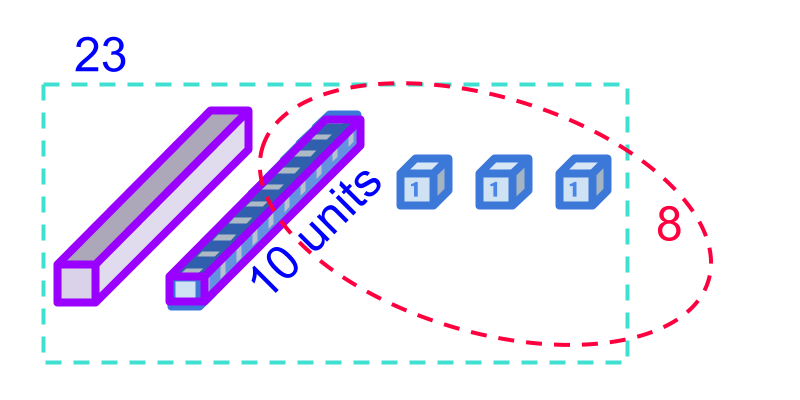A tens block is split into $10$$10$ units blocks. This is referred as de-grouping. The grouped place-value is degrouped into lower place-value.

De-grouping: When handling grouped numbers, at a place-value, $1$$1$ of a higher place-value can be de-grouped into $10$$10$ of the next lower place-value.

simplify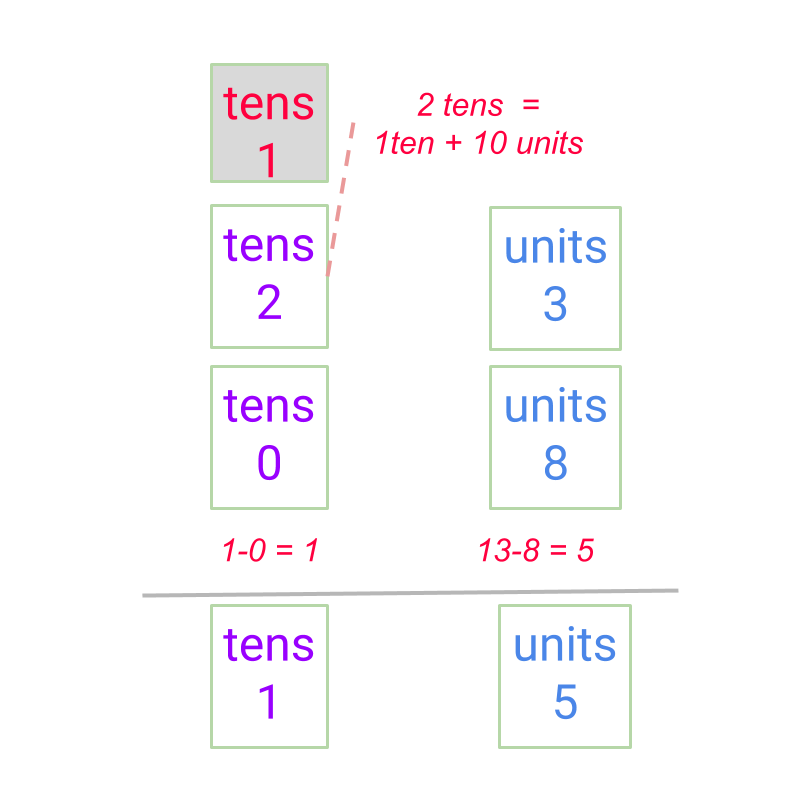Considering the subtraction $23-8$$23 - 8$. A simplified procedure, Subtraction by Place-value with de-grouping is shown in the figure.

$23-8$$23 - 8$ is considered.

In the units place minuend $3$$3$ is smaller than subtrahend $8$$8$. So, one ten is broken into $10$$10$ units. The units place subtraction is $13-8=5$$13 - 8 = 5$.

In the tens place, the subtraction is with remaining $1$$1$ ten in minuend as $1-0=0$$1 - 0 = 0$.

The difference is calculated as $15$$15$.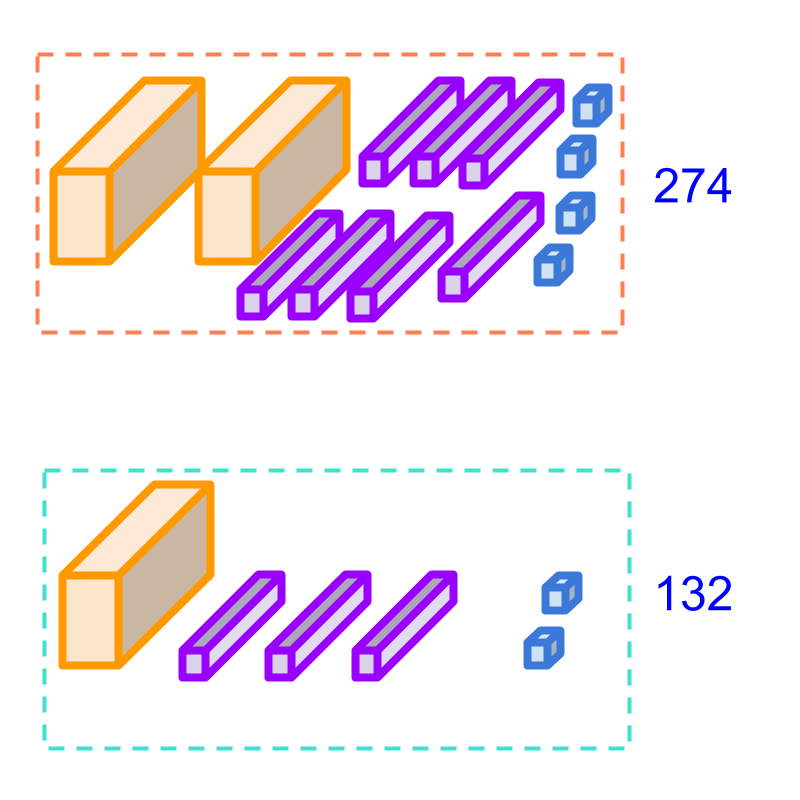Consider the subtraction of $274-132$$274 - 132$. We can quickly carryout the subtraction by the quantities in the figure. The difference is $142$$142$.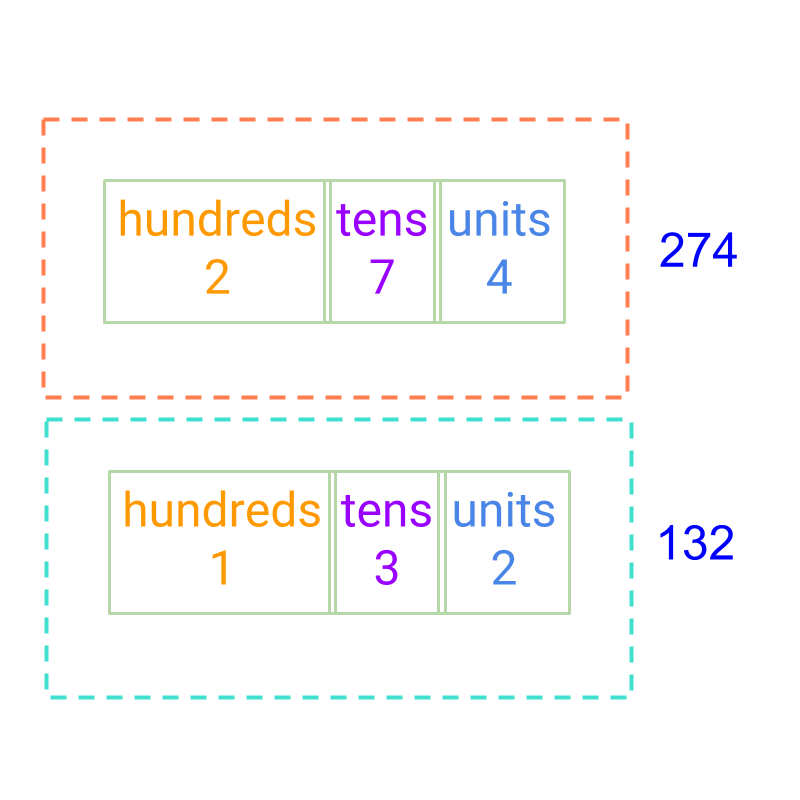Consider the subtraction $274-132$$274 - 132$. The numbers are given in place value in the figure. The difference is to be calculated.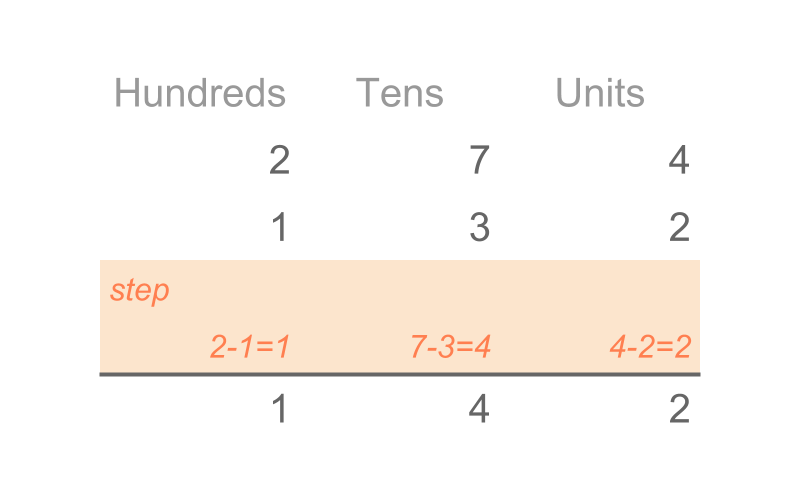Considering the subtraction $274-132$$274 - 132$.
A simplified procedure, Subtraction by Place-value with de-grouping is given in the figure.

The digits in the units place are subtracted,
Then the digits in the tens place are subtracted.
Then the digits in the hundreds place are subtracted.

The difference is $142$$142$.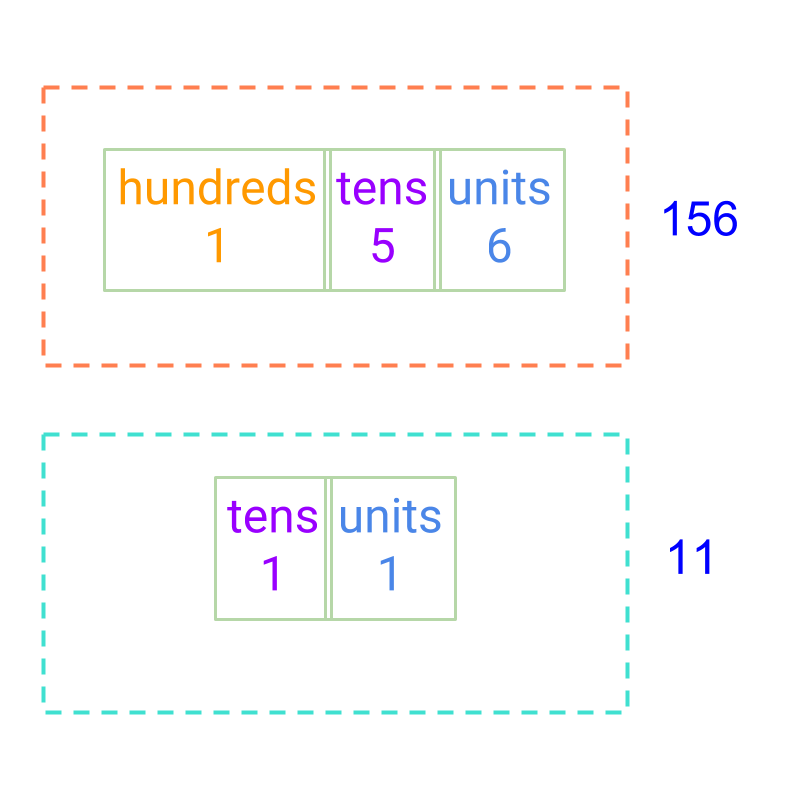Consider the subtraction $156-11$$156 - 11$. The answer is not $46$$46$. The correct answer is $145$$145$. Note that the units are subtracted from units, and the tens are subtracted from the tens.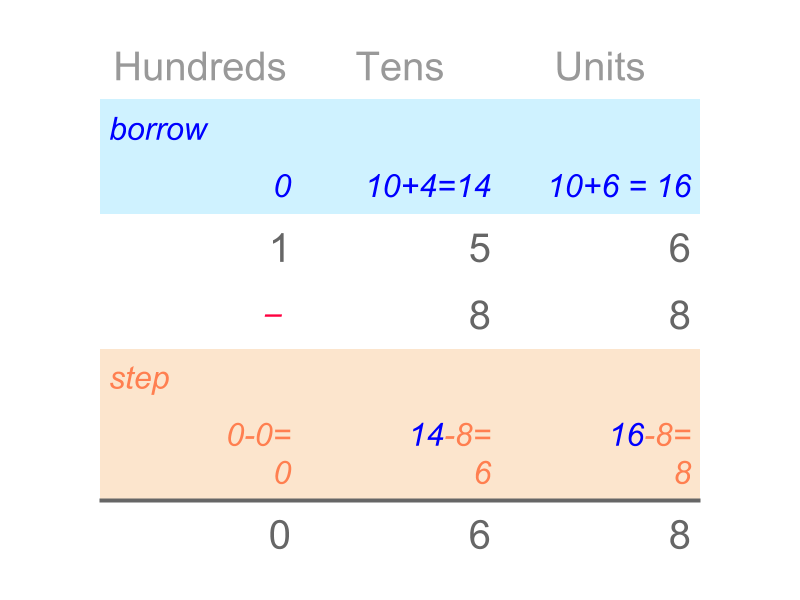Consider the subtraction $156-88$$156 - 88$. The simplified procedure, subtraction by place-value with de-grouping, is given in the figure.

The digits in units place are subtracted. Since $6$$6$ minuend is smaller than $8$$8$ subtrahend, the one ten from tens place is borrowed as $10$$10$ units into units place. The borrowed $10$$10$ units and the units in the minuend $6$$6$ together make $16$$16$.

Subtraction at units place is $16-8=8$$16 - 8 = 8$.

The digits in the tens place are subtracted. Since one ten was borrowed into the units place, the tens place of minuend is revised to $4$$4$. The $4$$4$ is less than the subtrahend $8$$8$. So, one hundred from hundreds place is borrowed as $10$$10$ tens. The borrowed $10$$10$ tens and the $4$$4$ tens in the minuend together make $14$$14$.

Subtraction at the tens place is $14-8=6$$14 - 8 = 6$.

Subtraction in the hundreds place is $0-0=0$$0 - 0 = 0$.

The difference is $68$$68$.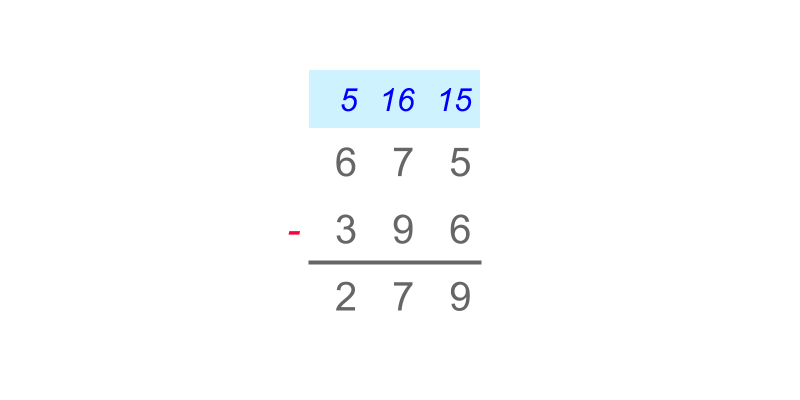Consider the subtraction $675-396$$675 - 396$. The simplified procedure is given in the figure. Note the subtraction of place values and the borrow are highlighted in color. The difference is $279$$279$.Subtraction by Place-value with de-grouping -- Simplified Procedure : Two numbers are subtracted as follows:

•  the place-value positions are arranged units under units, $10$$10$s under $10$$10$s, etc.

•  the units are subtracted and if the minuend digit is smaller than the subtrahend digit, then one ten's place is borrowed to make the minuend at the position larger. Then subtraction is carried out.

•  continue to the higher place-value position.

Note: The "borrow" is the simplification of taking $1$$1$ from a place value to $10$$10$ pieces of a lower place value.

examples

What is the difference $2332-1297$$2332 - 1297$ ?
The answer is "$1035$$1035$"

What is the difference $122-99$$122 - 99$?
The answer is "$23$$23$"

summary

Subtraction by Place-value with de-grouping -- Simplified Procedure : Two numbers are subtracted as follows:

•  the place-value positions are arranged units under units, $10$$10$s under $10$$10$s, etc.

•  the units are subtracted and if the minuend digit is smaller than the subtrahend digit, then one ten's place is borrowed to make the minuend at the position larger. Then subtraction is carried out.

•  continue to the higher place-value position.The "borrow" is the simplification of taking $1$$1$ from a place value to $10$$10$ pieces of a lower place value.

Outline